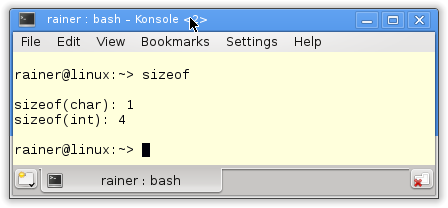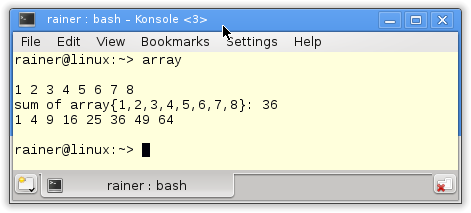# std::array – Dynamic Memory, no Thanks

std::array combines the best of two worlds. On the one hand, std::array has the size and efficiency of a C array; on the other hand, std::array has the interface of a std::vector.

std::array has a unique characteristic among all sequential containers of the Standard Template Library. You can not adjust its size during runtime. There are special rules for its initialization.

## The initialization

You have to keep the rule for aggregate initialization in mind:

• std::array<int,10> arr: The ten elements are not initialized.
• std::array<int,10>arr{}. The ten elements are value-initialized.
• std::array<int,10>arr{1,2,3,4): The remaining elements are value-initialized.

As a sequential container, std::array supports index access.

## Index access

std::array arr supports the index access in three ways.

• arr[n-1]: Access to the nth element without a check of the array boundaries.
• arr.at(n-1): Access the nth element by checking the array boundaries. Eventually, a std::range_error exception is thrown.
• std::get<n-1>(arr): Access the nth element by checking the array boundaries at compile time. The syntax is according to std::tuple.

std::get<n>(arr) shows the relationship of std::array with std::tuplestd::array is a homogeneous container of fixed size; std::tuple is a heterogeneous container of fixed size.

##Modernes C++ Mentoring

Be part of my mentoring programs:

• "Fundamentals for C++ Professionals" (open)
• "Design Patterns and Architectural Patterns with C++" (open)
• "C++20: Get the Details" (reopens December 2023)
• Do you want to stay informed about my mentoring programs: Subscribe via E-Mail.

I claimed that the C++ array is as memory efficient as a C array. The proof is still missing.

## Memory efficiency

My small program compares the memory efficiency of a C array, a C++ array, and a std::vector.

 ``` 1 2 3 4 5 6 7 8 9 10 11 12 13 14 15 16 17 18 19 20 21 22 23 24 25 26 27 28 29 30 31 32``` ```// sizeof.cpp #include #include #include int main(){ std::cout << std::endl; std::cout << "sizeof(int)= " << sizeof(int) << std::endl; std::cout << std::endl; int cArr= {1,2,3,4,5,6,7,8,9,10}; std::array cppArr={1,2,3,4,5,6,7,8,9,10}; std::vector cppVec={1,2,3,4,5,6,7,8,9,10}; std::cout << "sizeof(cArr)= " << sizeof(cArr) << std::endl; std::cout << "sizeof(cppArr)= " << sizeof(cppArr) << std::endl; std::cout << "sizeof(cppVec) = " << sizeof(cppVec) + sizeof(int)*cppVec.capacity() << std::endl; std::cout << " = sizeof(cppVec): " << sizeof(cppVec) << std::endl; std::cout << " + sizeof(int)* cppVec.capacity(): " << sizeof(int)* cppVec.capacity() << std::endl; std::cout << std::endl; } ```

The numbers speak a clear language.Both the C array (line 22) and the C++ array (line 24) take 40 bytes. That is precisely sizeof(int)*10. In opposite to them, std::vector needs additional 24 bytes (line 27) to manage its data on the heap. cppVec.capacity() is the number of elements a std::vector cppVec can have without acquiring new memory. I described the details of the memory management of std::vector and std::string in the post Automatic memory management of the STL containers.

Before I complete the picture and show the example, I want to emphasize it explicitly: The great value of a std::array in opposition to a  C array is that std::array knows it size.

## std::array in action

One additional value of a std::array compared to a C array is that a std::array feels like a std::vector.

 ``` 1 2 3 4 5 6 7 8 9 10 11 12 13 14 15 16 17 18 19 20 21 22 23 24 25 26 27 28 29``` ```// array.cpp #include #include #include int main(){ std::cout << std::endl; // output the array std::array array1{1,2,3,4,5,6,7,8}; std::for_each( array1.begin(),array1.end(),[](int v){std::cout << v << " ";}); std::cout << std::endl; // calculate the sum of the array by using a global variable int sum = 0; std::for_each(array1.begin(), array1.end(),[&sum](int v) { sum += v; }); std::cout << "sum of array{1,2,3,4,5,6,7,8}: " << sum << std::endl; // change each array element to the second power std::for_each(array1.begin(), array1.end(),[](int& v) { v=v*v; }); std::for_each( array1.begin(),array1.end(),[](int v){std::cout << v << " ";}); std::cout << std::endl; std::cout << std::endl; } ```

Therefore, you can output array1 in line 13 with a lambda function and the range-based for-loop. Using the summation variable sum in line 19, you can sum up the elements of the std::array. The lambda function in line 23 takes its arguments by reference and can therefore map each element to its square. Nothing special, but we are dealing with a std::array.

And here is the output of the program.### For clarification

With C++11, we have the free function templates std::begin and std::end returning iterators for a C array. So a C array is quite comfortable and safe to use with these function templates because you do have not to remember its size.

 ``` 1 2 3 4 5 6 7 8 9 10 11 12 13 14 15 16 17 18 19 20 21 22 23 24 25 26 27 28``` ```// cArray.cpp #include #include int main(){ std::cout << std::endl; // output the array int array1[] = { 1, 2, 3, 4, 5, 6 ,7, 8}; std::for_each( std::begin(array1), std::end(array1), [](int v){ std::cout << v << " "; }); std::cout << std::endl; // calculate the sum of the array by using a global variable int sum = 0; std::for_each(std::begin(array1), std::end(array1), [&sum](int v) { sum += v; }); std::cout << "sum of array{1, 2, 3, 4, 5, 6, 7, 8}: " << sum << std::endl; // change each array element to the second power std::for_each(std::begin(array1), std::end(array1), [](int& v) { v=v*v; }); std::for_each(std::begin(array1), std::end(array1), [](int v){ std::cout << v << " "; }); std::cout << std::endl; std::cout << std::endl; } ```

Of course, the result is the same.

## What’s next?

This post was concise. In the next post, I will look closely at one of the prominent C++11 features: move semantic.

Thanks a lot to my Patreon Supporters: Matt Braun, Roman Postanciuc, Tobias Zindl, G Prvulovic, Reinhold Dröge, Abernitzke, Frank Grimm, Sakib, Broeserl, António Pina, Sergey Agafyin, Андрей Бурмистров, Jake, GS, Lawton Shoemake, Jozo Leko, John Breland, Venkat Nandam, Jose Francisco, Douglas Tinkham, Kuchlong Kuchlong, Robert Blanch, Truels Wissneth, Kris Kafka, Mario Luoni, Friedrich Huber, lennonli, Pramod Tikare Muralidhara, Peter Ware, Daniel Hufschläger, Alessandro Pezzato, Bob Perry, Satish Vangipuram, Andi Ireland, Richard Ohnemus, Michael Dunsky, Leo Goodstadt, John Wiederhirn, Yacob Cohen-Arazi, Florian Tischler, Robin Furness, Michael Young, Holger Detering, Bernd Mühlhaus, Matthieu Bolt, Stephen Kelley, Kyle Dean, Tusar Palauri, Dmitry Farberov, Juan Dent, George Liao, Daniel Ceperley, Jon T Hess, Stephen Totten, Wolfgang Fütterer, Matthias Grün, Phillip Diekmann, Ben Atakora, Ann Shatoff, Rob North, Bhavith C Achar, and Marco Parri Empoli.

Thanks, in particular, to Jon Hess, Lakshman, Christian Wittenhorst, Sherhy Pyton, Dendi Suhubdy, Sudhakar Belagurusamy, Richard Sargeant, Rusty Fleming, John Nebel, Mipko, Alicja Kaminska, Slavko Radman, and David Poole.

 My special thanks to EmbarcaderoMy special thanks to PVS-StudioMy special thanks to Tipi.buildMy special thanks to Take Up Code## Seminars

I’m happy to give online seminars or face-to-face seminars worldwide. Please call me if you have any questions.

### Standard Seminars (English/German)

Here is a compilation of my standard seminars. These seminars are only meant to give you a first orientation.

• C++ – The Core Language
• C++ – The Standard Library
• C++ – Compact
• C++11 and C++14
• Concurrency with Modern C++
• Design Pattern and Architectural Pattern with C++
• Embedded Programming with Modern C++
• Generic Programming (Templates) with C++

#### New

• Clean Code with Modern C++
• C++20

### Contact Me

Modernes C++ Mentoring,Tags:
0 replies